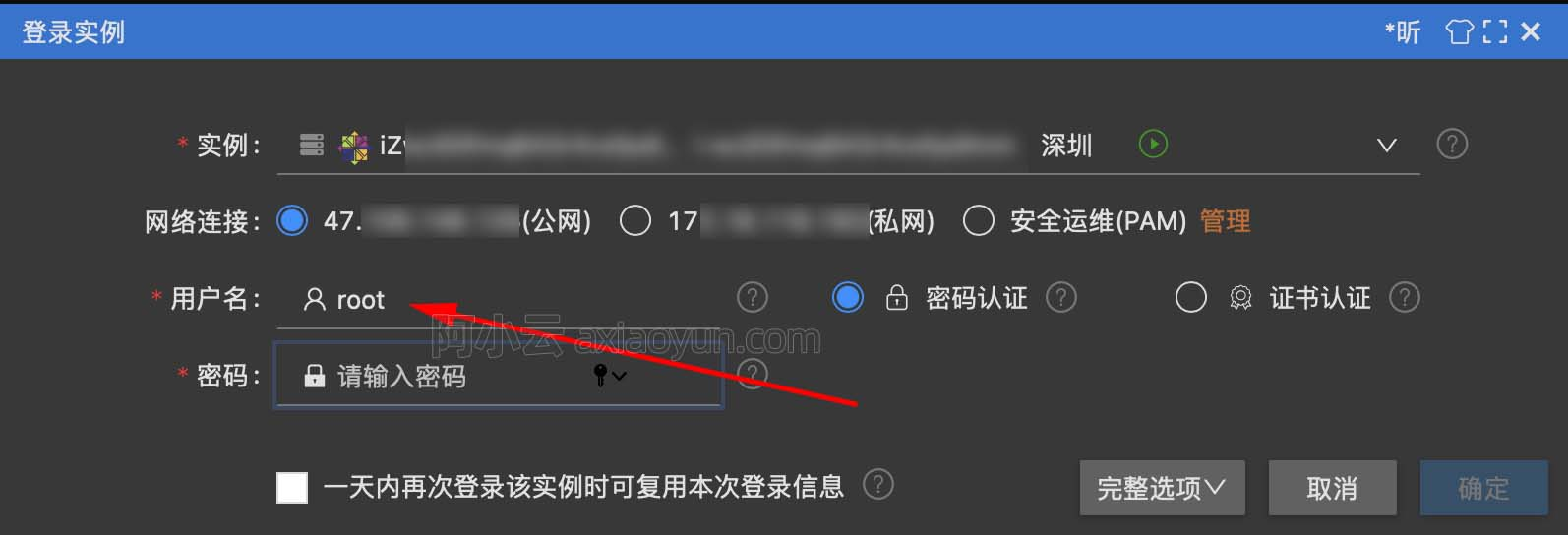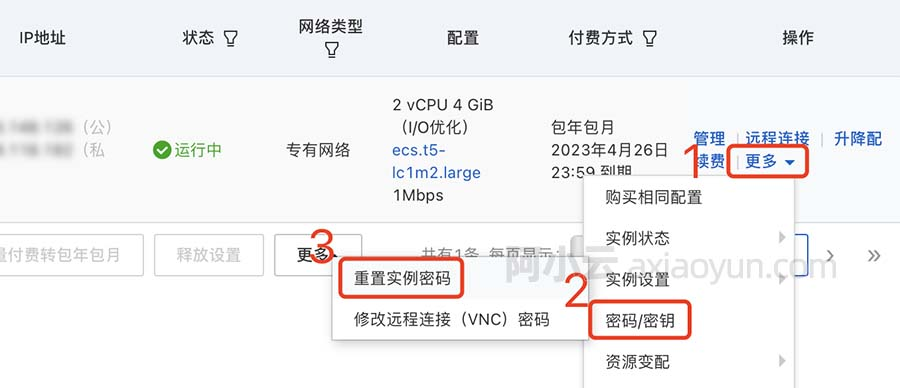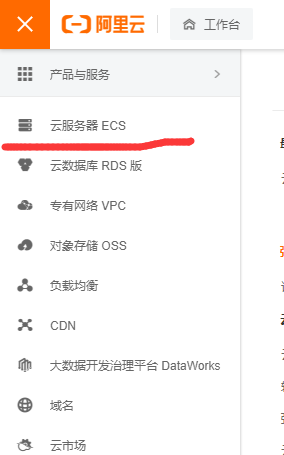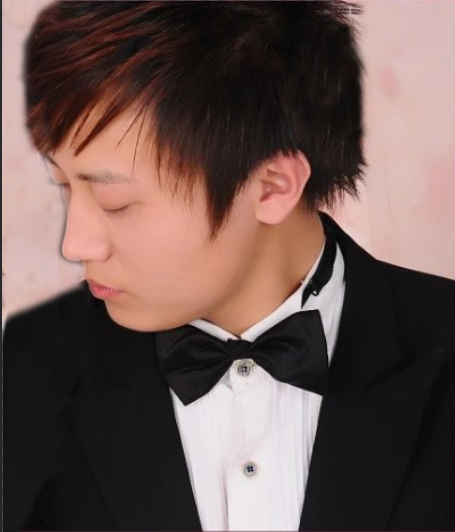# 浅析Python的类、继承和多态

+关注继续查看

1. # point.py
2.
3. class Point:
4.
5.     def __init__(self, x=0, y=0):
6.
7.         self.x, self.y = x, y

1. >>> from point import *
2.
3. >>> p = Point(10, 10) # __init__ 被调用
4.
5. >>> type(p)
6.
7. <class 'point.Point'
8.
9. >>> p.x, p.y
10.
11. (10, 10)

1. >>> type(Point)
2.
3. <class 'type'
4.
5. >>> dir(Point)
6.
7. ['__class__''__delattr__''__dict__', ..., '__init__', ...]
8.
9. >>> Point.__class__
10.
11. <class 'type'>

Point 是 type 的一个实例，这和 p 是 Point 的一个实例是一回事。

1. class Point:
2.
3.     ...
4.
5.     def set(self, x, y):
6.
7.         self.x, self.y = x, y

1. >>> p = Point(10, 10)
2.
3. >>> p.set(0, 0)
4.
5. >>> p.x, p.y
6.
7. (0, 0)

p.set(...) 其实只是一个语法糖，你也可以写成 Point.set(p, ...)，这样就能明显看出 p 就是 self 参数了：

1. >>> Point.set(p, 0, 0)
2.
3. >>> p.x, p.y
4.
5. (0, 0)

1. class Point:
2.
3. ...
4.
5. def set(this, x, y):
6.
7. this.x, this.y = x, y

Python 没有 public / protected / private 这样的访问控制，如果你非要表示“私有”，习惯是加双下划线前缀。

1. class Point:
2.
3.     def __init__(self, x=0, y=0):
4.
5.         self.__x, self.__y = x, y
6.
7.
8.
9.     def set(self, x, y):
10.
11.         self.__x, self.__y = x, y
12.
13.
14.
15.     def __f(self):
16.
17.         pass

__x、__y 和 __f 就相当于私有了：

1. >>> p = Point(10, 10)
2.
3. >>> p.__x
4.
5. ...
6.
7. AttributeError: 'Point' object has no attribute '__x'
8.
9. >>> p.__f()
10.
11. ...
12.
13. AttributeError: 'Point' object has no attribute '__f'

_repr_

1. >>> p = Point(10, 10)
2.
3. >>> p
4.
5. <point.Point object at 0x000000000272AA20>

1. >>> p
2.
3. Point(10, 10)

1. class Point:
2.
3. def __repr__(self):
4.
5. return 'Point({}, {})'.format(self.__x, self.__y)

1. >>> repr(p)
2.
3. 'Point(10, 10)'

_str_

1. class Point:
2.
3. def __str__(self):
4.
5. return '({}, {})'.format(self.__x, self.__y)

1. >>> p1 = Point(10, 10)
2.
3. >>> p2 = Point(10, 10)
4.
5. >>> p3 = p1 + p2
6.
7. Traceback (most recent call last):
8.
9. File "<stdin>", line 1, in <module>
10.
11. TypeError: unsupported operand type(s) for +: 'Point' and 'Point'

1. class Point:
2.
4.
5. return Point(self.__x + other.__x, self.__y + other.__y)

1. >>> p3 = p1 + p2
2.
3. >>> p3
4.
5. Point(20, 20)

Python 的内建类型，比如字符串、列表，都“重载”了 + 操作符。

1. # shape.py
2.
3.
4.
5. class Shape:
6.
7.     def area(self):
8.
9.         return 0.0
10.
11.
12.
13. class Circle(Shape):
14.
15.     def __init__(self, r=0.0):
16.
17.         self.r = r
18.
19.
20.
21.     def area(self):
22.
23.         return math.pi * self.r * self.r
24.
25.
26.
27. class Rectangle(Shape):
28.
29.     def __init__(self, a, b):
30.
31.         self.a, self.b = a, b
32.
33.
34.
35.     def area(self):
36.
37.         return self.a * self.b

1. >>> from shape import *
2.
3. >>> circle = Circle(3.0)
4.
5. >>> circle.area()
6.
7. 28.274333882308138
8.
9. >>> rectangle = Rectangle(2.0, 3.0)
10.
11. >>> rectangle.area()
12.
13. 6.0

1. class Circle(Shape):
2.
3. pass

1. >>> Shape.area is Circle.area
2.
3. True

1. >>> from shape import *
2.
3. >>> Shape.area is Circle.area
4.
5. False

1. >>> Shape.__dict__['area'
2.
3. <function Shape.area at 0x0000000001FDB9D8>
4.
5. >>> Circle.__dict__['area'
6.
7. <function Circle.area at 0x0000000001FDBB70>

1. class Circle(Shape):
2.
3. ...
4.
5. # def area(self):
6.
7. return math.pi * self.r * self.r
8.
9. # 为 Circle 添加 area 方法。
10.
11. Circle.area = lambda self: math.pi * self.r * self.r

Python 官方教程「9. Classes」第一句就是：

Compared with other programming languages, Python’s class mechanism adds classes with a minimum of new syntax and semantics.

Python 以最少的新的语法和语义实现了类机制，这一点确实让人惊叹，但是也让 C++ / Java 程序员感到颇为不适。

1. class AbstractEventLoop:
2.
3. def run_forever(self):
4.
5. raise NotImplementedError
6.
7. ...

1. loop = asyncio.AbstractEventLoop()
2.
3. try:
4.
5. loop.run_forever()
6.
7. except NotImplementedError:
8.
9. pass

C++ 可以通过纯虚函数或设构造函数为 protected 来避免接口被实例化，Java 就更不用说了，接口就是接口，有完整的语法支持。

1. class AbstractShape:
2.
3. def area(self):
4.
5. raise NotImplementedError

super

1. class AbstractShape:
2.
3. def __init__(self, color):
4.
5. self.color = color

1. class Circle(AbstractShape):
2.
3. def __init__(self, color, r=0.0):
4.
5. super().__init__(color)
6.
7. self.r = r

1. class Circle(AbstractShape):
2.
3. def __init__(self, color, r=0.0):
4.
5. AbstractShape.__init__(self, color)
6.
7. self.r = r20724 017265 024766 014042 014926 019835 022473 014845 023013 015990 0

17112

2569

JS零基础入门教程（上册）